# How to use MAX function in Microsoft Excel

In this article, we’ll learn about MAX function in Microsoft Excel.

In Excel, MAX function is used to find the largest or maximum number from a range of cells.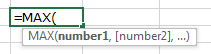With the help of examples, let us understand the use of MAX function in Excel.

Refer to these 5 different examples for the usage of MAX function in Microsoft Excel on the basis of following sample data: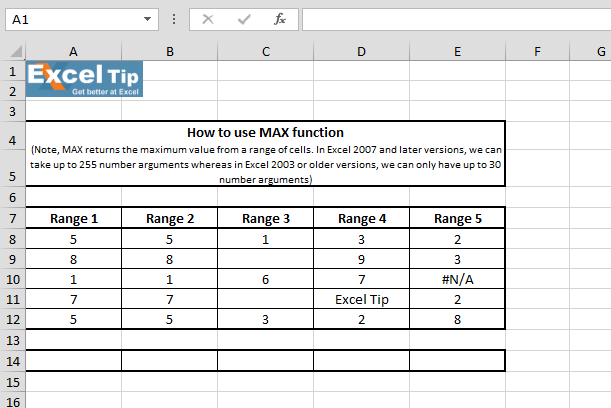1st Example:-

We have a range of numbers from which we want to retrieve maximum numbers.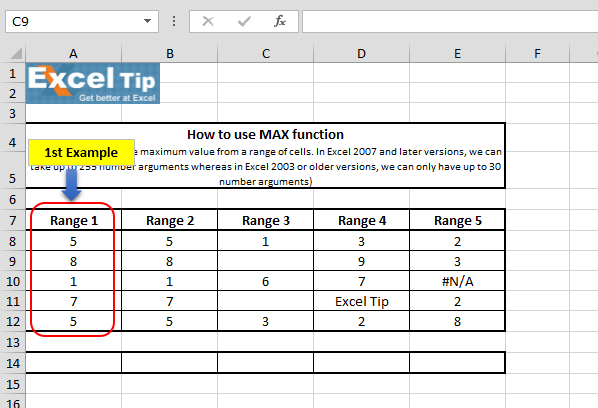Follow below given steps:-

• Enter the function in cell A14
• =MAX(A8:A12)
• Press Enter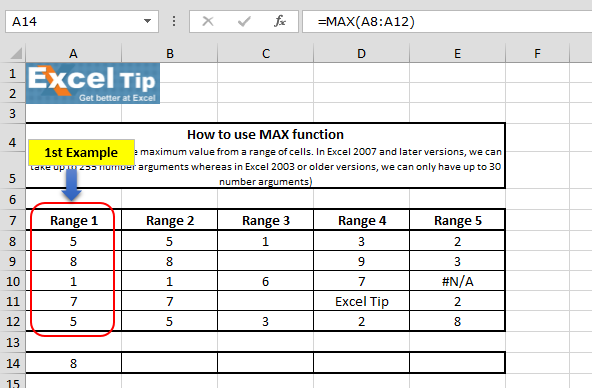2nd Example:-

In this example let’s see what happens when we enter some value in the function to along with the range.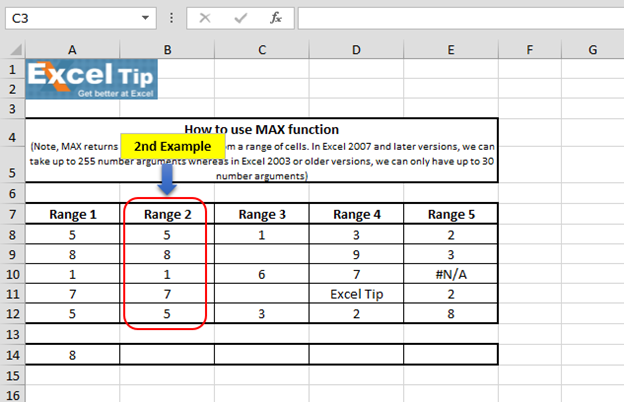Follow the steps given below:-

• Enter the function in cell B14
• =MAX(B8:B12,15,5*4)
• Press Enter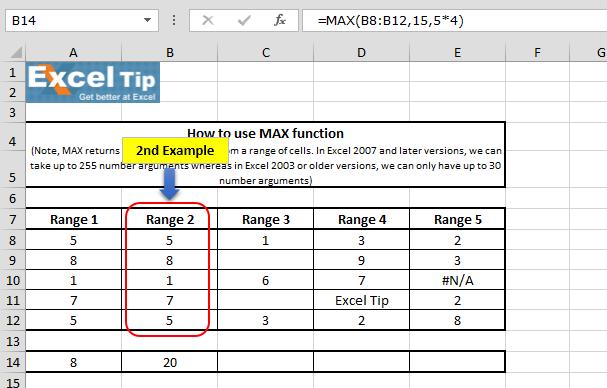• Function has returned 20 and in the third argument we have entered 4*5 which is equal to 20 which is the largest value amongst the range and the logical values

3rd Example:-

In this example, we will refer to the blank cells in the function range.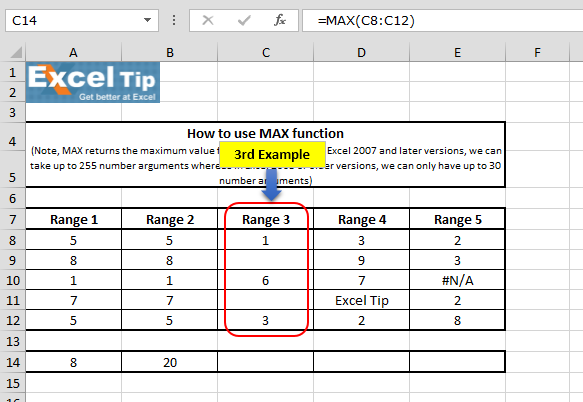Follow the steps given below:-

• Same, enter the Max function in cell
• =MAX(C8:C12)
• Press Enter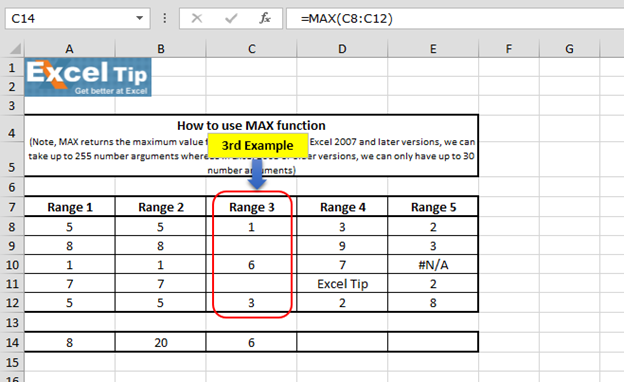By ignoring the blank cells, Max function returns the result on the basis of available value within the range.

4th Example:-

In this example, we will see what happens when text is available within the range.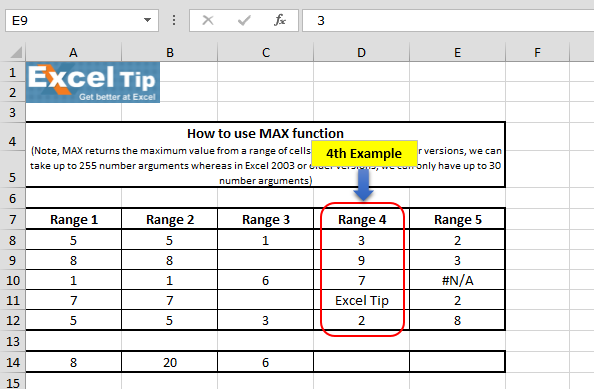Follow the steps given below:-

• Enter the function in cell D14
• =MAX(D8:D12)
• Press Enter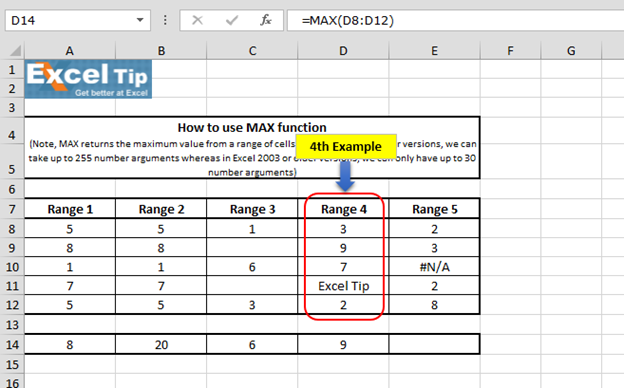We can see in the above image the function has returned maximum available value within defined range. It means MAX function ignores cell that contains text within specified range.

5th Example:-

In this example, we have taken error value within range.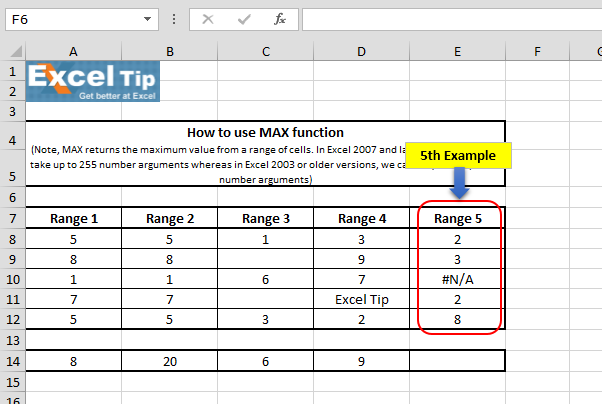Follow the steps given below:-

• Enter the function in cell E14
• =MAX(E8:E12)
• Press Enter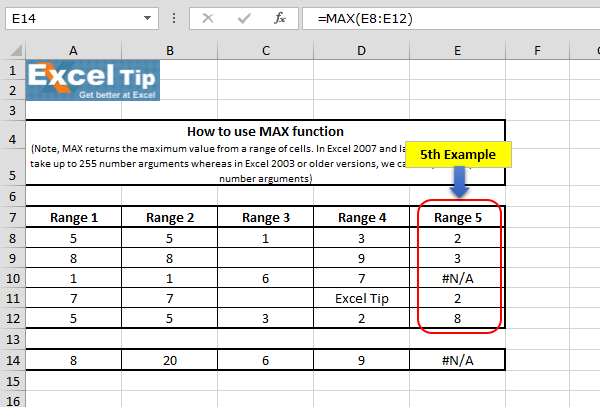Function has returned the same NA error because it cannot have any error value in the range which we are referring to in the number argument and hence it returns an error as an answer.

So, this is how MAX function works and we can make use of it in different situations.# Video: How to use MAX function in Microsoft Excel

Watch the steps in this short video, and the written instructions are above the video

If you liked our blogs, share it with your friends on Facebook. And also you can follow us on Twitter and Facebook.
We would love to hear from you, do let us know how we can improve, complement or innovate our work and make it better for you. Write us at info@exceltip.com

Terms and Conditions of use

The applications/code on this site are distributed as is and without warranties or liability. In no event shall the owner of the copyrights, or the authors of the applications/code be liable for any loss of profit, any problems or any damage resulting from the use or evaluation of the applications/code.